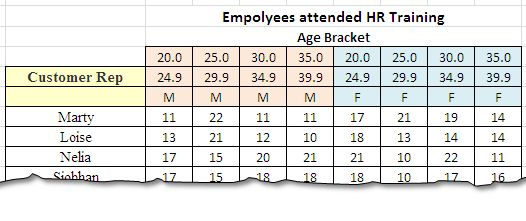# Using SUMPRODUCT() To Validate Multiple Criteria In ColumnsWe have studied and used SUMIFS() and SUMPRODUCTS() function with their appropriates use. Just to recall, SUMIFS() work great when our data is in the shape of list or is in column. We can use them to our convenience. But when the data is in set in rows, SUMIFS() does not work and we have to revert to SUMPRODUCT() that is more robust for such cases.

SUMPRODUCT() has the ability to validate the criteria for both rows and column. Basically it is an array formula and we use is virtue of treating data as array to validate it for any criteria.

Our today’s post is related to validating criteria’s in the header row of the data – be it a single row or set of rows, we will learn how to use SUMPRODUCT() for this purpose.

As usual we will take an example with some sample data to proceed.

### Sample Data

The following picture shows the sample data to be used for this post. We have name of employees on the left most side where as the header row contains age brackets. The table is divided into two parts – male and females with different age brackets.### Our Question

How many males between 25 and 35 have attended the training by Customer Representative Loise?

We will start by validating criteria in columns ad in rows. The formula that should work will be:

=SUMPRODUCT((\$C\$6:\$J\$6=C6)*(\$B\$7:\$B\$14=\$B\$8)*(\$C\$4:\$J\$4>=D4)*(\$C\$4:\$J\$4<=E4)*(\$C\$7:\$J\$14))

Lets start examining this formula part by part:Part # 1

The part (\$C\$6:\$J\$6=C6) examines the ranges C6:J6 if they are males by checking the values in the row for “M”. If a match comes positive, a 1 is returned as a result otherwise we get a zero.

Part # 2

This part (\$B\$7:\$B\$14=B8) validates the name of the trainer. According to the requirement, if the name comes to be Loise, the array will return a 1 and other wise a zero will be returned.

Part # 3 and Part 4

These two arguments actually validates if the age is greater then or equal to 25 and less then or equal to 30 using the logical operators “>=” and “<=”. Once done with the matching the arrays multiply to give the final result.

Part # 5

This is the actual body of the data table that contains the values. The arrays from all of the four validations are multiplied to give a final sum and the result is returned in the respective cell.